V18/7/14

## Dose Assessment for Building Products¶

### CEN TC 351 WG3 TG32¶

This is an impementation in R  of the dose estimation for building materials due to natural radionuclides. It's based on a methode used in an internal report of the finish office for radiation protection STUK , and was afterward the base of the famous index formula of the publication Radiation Protection 112  by the European Commission.

The annual dose is estimated by a point kernel methode using Berger coefficients. The parameters were choosen to be compatible to the RP112, but the room size was adapted from the room used by CEN TC351 WG2 (without door and window).

 R Development Core Team (2008). R: A language and environment for statistical computing. R Foundation for Statistical Computing, Vienna, Austria. ISBN 3-900051-07-0, URL http://www.R-project.org. The script was tested with R version 3.5.0 (Joy in Playing).

 Markkanen M. Radiation Dose Assessments for Materials with Elevated Natural Radioactivity. Report STUK-B-STO 32, Radiation and Nuclear Safety Authority – STUK, 1995.

 RP112: Radiological Protection Principles concerning the Natural Radioactivity of Building Materials

Remarks:

a) The absorption coefficient is scaled linear (as an approxiamtion) with the density of the material.

b) Here, the mean gamma energy for Radium is 0.810 MeV as used in RP112. The correct value is 0.860 MeV.

c) In RP112, the coefficient to harmonise the units was 5.77E-7. Here, it is 5.77E-10. It's still a open question, where's the difference come from. but nevertheless, the results of the calculations in the RP112 are correct.

A library which includs a funktion for numerical solution of 3d integrals, has to be loaded. (https://cran.r-project.org/package=cubature) This script was tested with V1.3-11.

In :
library(cubature)


First, a fuction "walldose" with the width and height of the wall (cm), the distance from the wall (cm) as well as the thickness (cm) and density (kg/cm$^3$) is defined. The result is the annual dose per activity concentration in mSv/(Bq/kg) for radium, thorium and potassium separatelly.

Second, a function "roomdose" can be defined, wich add the dose of all six walls with the size of the WG2-room. Fixpoint is the center of the room. The free parameters are the thickness (cm) and the density (kg/cm$^3$) of the walls. Still, the result is the annual dose per activity concentration in mSv/(Bq/kg) for radium, thorium and potassium separatelly.

In :
walldose <- function(x,y,z,d,rho) {
gam <- c(2.12, 2.05, 0.356, 0.107) # emission probability
mue <- c(0.166, 0.193, 0.0927, 0.124)*rho/2.350 # linear absorption coefficient of concrete, density corrected, 1/cm
muer <- c(0.0285, 0.0295, 0.0217, 0.0257) # linear absorption coefficient of air, 10^(-5) cm^2/g
Cbe <- c(1.161, 1.279, 0.734, 0.946) # Berger coefficient
Dbe <- c(0.144, 0.190, 0.0234, 0.0755) # Berger coefficient
E <- c(0.810, 0.587, 2.615, 1.461) # # average gamma energies in MeV 0.810 -> 0.860?
conc <- c(1,1,1,1) # Activity oncentration, normalized to 1 Bq/kg
wall <- c(x,y,d)
xp <- c(0,0,z) # Point at the middle of the wall at a distance

itegr <-c(0,0,0,0)

l <- function(x) {sqrt((xp-x)^2+(xp-x)^2+(xp-x)^2)}
s <- function(x) {abs(x/(xp-x))*l(x)}

inte1 <- function(x) {
(1+Cbe*mue*s(x)*exp(Dbe*mue*s(x)))*exp(-mue*s(x))/l(x)^2}
inte2 <- function(x) {
(1+Cbe*mue*s(x)*exp(Dbe*mue*s(x)))*exp(-mue*s(x))/l(x)^2}
inte3 <- function(x) {
(1+Cbe*mue*s(x)*exp(Dbe*mue*s(x)))*exp(-mue*s(x))/l(x)^2}
inte4 <- function(x) {
(1+Cbe*mue*s(x)*exp(Dbe*mue*s(x)))*exp(-mue*s(x))/l(x)^2}

itegr <- adaptIntegrate(inte1, c(-wall/2,-wall/2,-wall),c(wall/2,wall/2,0))$integral itegr <- adaptIntegrate(inte2, c(-wall/2,-wall/2,-wall),c(wall/2,wall/2,0))$integral
itegr <- adaptIntegrate(inte3, c(-wall/2,-wall/2,-wall),c(wall/2,wall/2,0))$integral itegr <- adaptIntegrate(inte4, c(-wall/2,-wall/2,-wall),c(wall/2,wall/2,0))$integral

dummy <- 0.7*7000*1000*(5.77e-10)/4/pi*rho*conc*gam*muer*E*itegr
Dose <- c(dummy, dummy+dummy,dummy)
Dose
}

In :
roomdose <- function(d,rho) {
w1 <- 2*walldose(400,300,125,d,rho)
w2 <- 2*walldose(400,250,150,d,rho)
w3 <- 2*walldose(300,250,200,d,rho)
w1+w2+w3
}


In a first test, the annual dose is calculated according to the first example of the TG32-report TR17113: Thickness 20 cm, density 2,350 kg/cm$^3$, C$_{Ra}$ = C$_{Th}$ = 80 Bq/kg, C$_{K}$ = 800 Bq/kg. A background of 0,29 mSv is considered as the population weighted average for Europe based on data of UNSCEAR.

In :
test = roomdose(20,2.350)

In :
(80*test+80*test+800*test)-0.29

0.767783407169158

The annual dose in addition to the background is therefore estimated to 0,77 mSv.

In a second test, the dependencies of the dose in relation to the thickness and to the density is estimated. in the first plot, the density of the Material is 2,350 kg/m$^3$ resp. 800 kg/cm$^3$ (dashed lines).

In :
N <- 50
q <- array(0, dim=c(N,3))
p <- array(0, dim=c(N,3))
x <- array(0, dim=c(N))
for (i in 1:N){x[i] <- i; q[i,] <- roomdose(i,2.350); p[i,] <- roomdose(i,0.800)}

plot(x, q[,1], main="eff. Dose in Model Room", xlab="Thickness (cm)",
ylab="mSv/a per Bq/kg", xlim=c(0,N), ylim=c(0,0.006), type="l", col="red")
lines(x,q[,2], col="black")
lines(x,q[,3], col="green")
lines(x,p[,1], col="red", lty  = "dashed")
lines(x,p[,2], col="black", lty  = "dashed")
lines(x,p[,3], col="green", lty  = "dashed")

legend("topleft", c("Ra","Th","K"), col=c('red','black','green'), lty=1, bty="n")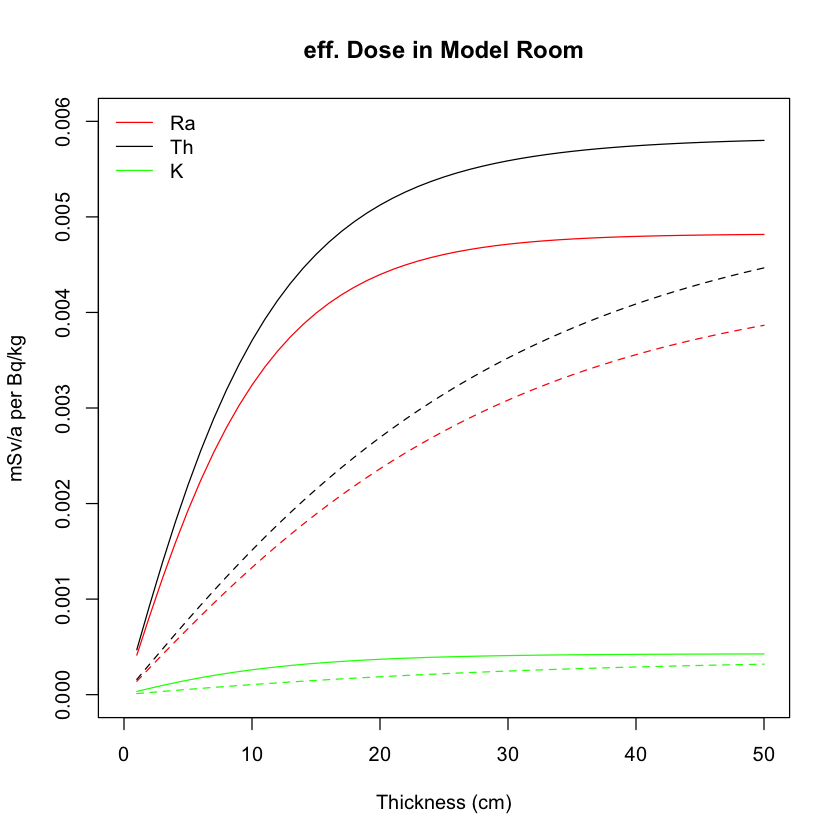Now, the thickness is fixed to 20 cm:

In :
N <- 50
q <- array(0, dim=c(N,3))
x <- array(0, dim=c(N))
for (i in 1:N){x[i]<-2.350*i/N; q[i,]<- roomdose(20,2.350*i/N)}
plot(x,q[,2], main="eff. Dose in Model Room",
xlab=expression("Density (kg/m"^3*")"),
ylab="mSv/a per Bq/kg", xlim=c(0,2.5), ylim=c(0,0.006), type="l")
lines(x,q[,1], col="red")
lines(x,q[,3], col="green")
legend("topleft", c("Ra", "Th", "K"), lty=1, col=c('red','black','green'), bty='n')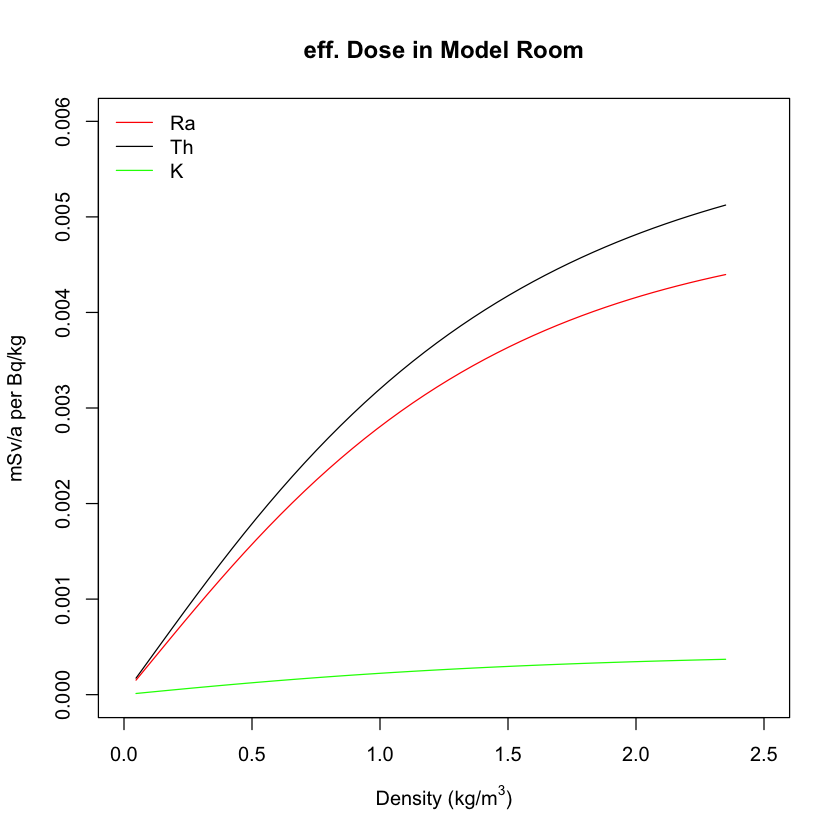From the plot, it can be evaluated that for most of the cases, a saturation can be assumed for values higher than ca. 20 cm in thickness and ca. 2.350 kg/cm$^3$ resp. ca. 50 kg/cm$^2$ for the mass per unit area.

The next step ist to combine the two dependencies into one contour plot.

The number of steps N can be choosen between about 20 and 50.

In :
n = 50 # Number of steps
Ra <- array(0, dim=c(n,n))
Th <- array(0, dim=c(n,n))
K <- array(0, dim=c(n,n))
dummy <- array(0, dim=c(3))

for (i in 1:n){
for (j in 1:n){
dummy <- roomdose(40*i/n,2.50*j/n)
Ra[i,j] <- dummy
Th[i,j] <- dummy
K[i,j] <- dummy
}
}

In :
q2 <- seq(0, 2.5, len = n)
q1 <- seq(0, 50, len = n)
image(q1, q2, Ra, col = terrain.colors(256), axes = TRUE,
main = "eff. Dose in Model Room, Ra, mSv/a per Bq/kg", xlab = "Thickness (cm)",
ylab=expression("Density (g/cm"^3*")"))
contour(q1, q2, Ra, levels = c(0.001,0.002,0.003,0.004), add = TRUE)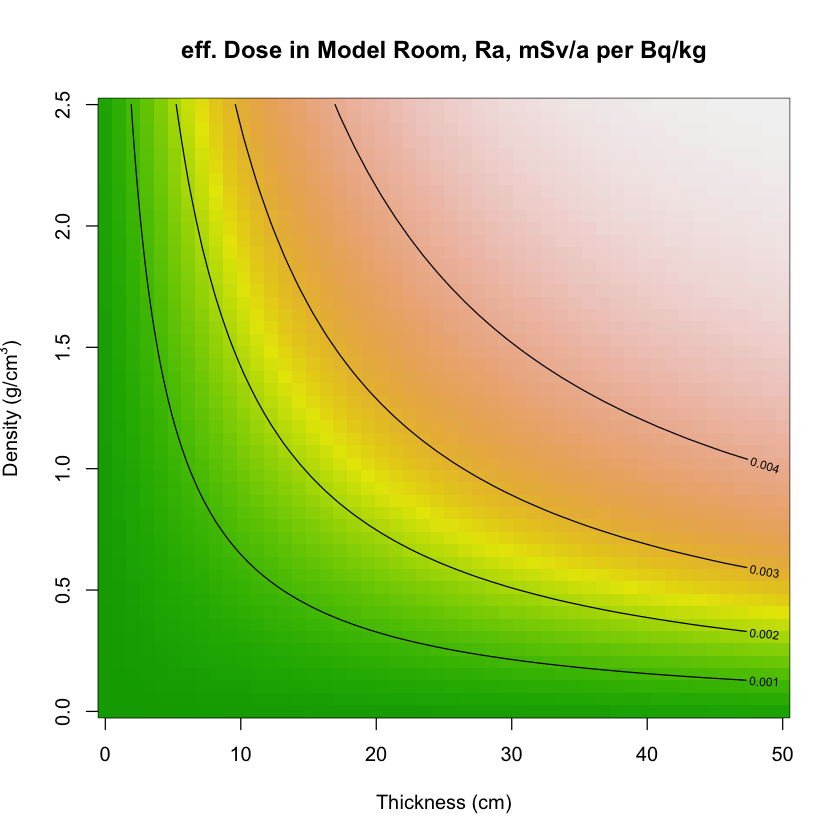In :
image(q1, q2, Th, col = terrain.colors(256), axes = TRUE,
main = "eff. Dose in Model Room, Th, mSv/a per Bq/kg", xlab = "Thickness (cm)",
ylab=expression("Density (g/cm"^3*")"))
contour(q1, q2, Th, levels = c(0.001,0.002,0.003,0.004), add = TRUE)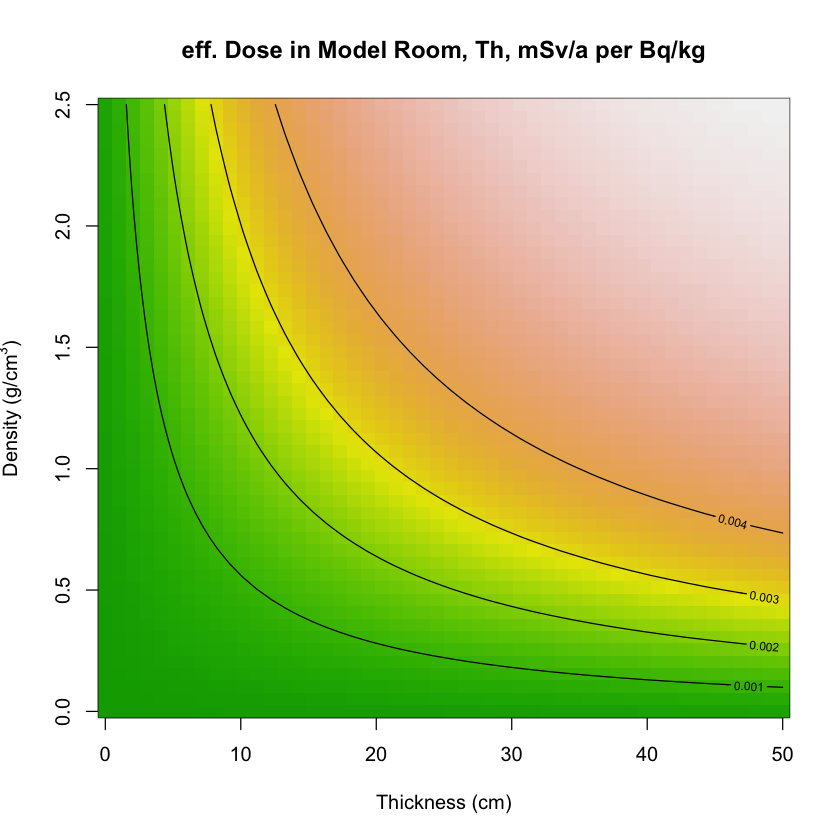In :
image(q1, q2, K, col = terrain.colors(256), axes = TRUE,
main = "eff. Dose in Model Room, K, mSv/a per Bq/kg", xlab = "Thickness (cm)",
ylab=expression("Density (g/cm"^3*")"))
contour(q1, q2, K, levels = c(0.0001,0.0002,0.0003,0.0004), add = TRUE)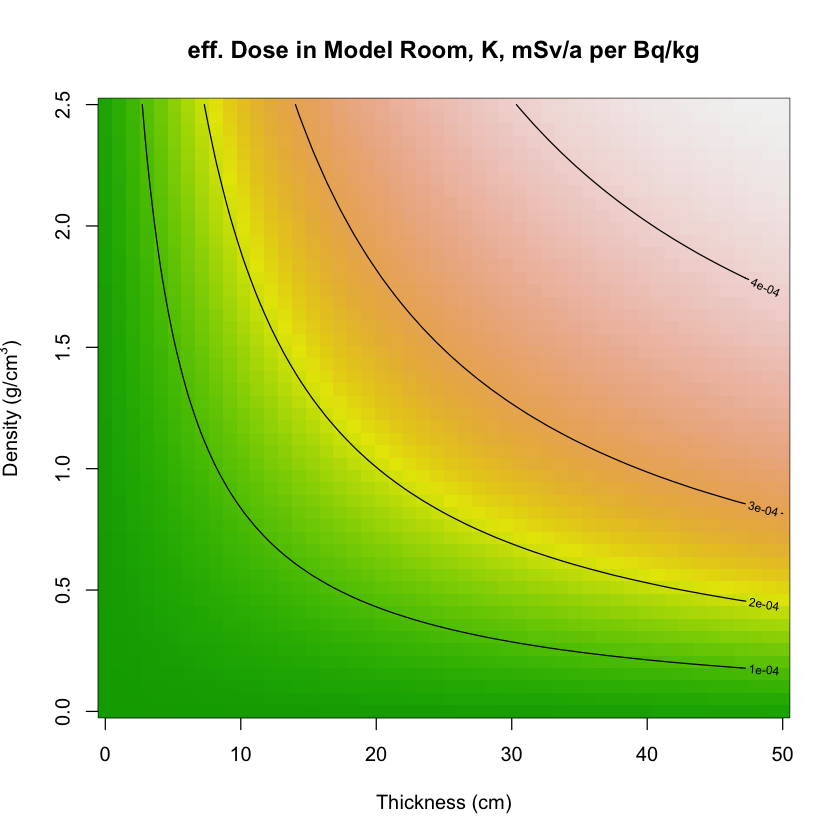The isolines look like rectangular hyperbola, witch propose that the dose is a function of the product of density and thickness (aka mass per unit area). To test this assumption, the dose is plotted for different thickness but constant masses per unit area. The isolines should be parallels.

In :
n <- 50
Ra_FD <- array(0, dim=c(n,n))
Th_FD <- array(0, dim=c(n,n))
K_FD <- array(0, dim=c(n,n))
dummy <- array(0, dim=c(3))

for (i in 1:n){
for (j in 1:n){
dummy <- roomdose(50*i/n,2.5*j/i)
Ra_FD[i,j] <- dummy
Th_FD[i,j] <- dummy
K_FD[i,j] <- dummy
}
}

In :
q1 <- seq(1, 50, len = n)
q2 <- seq(1, 125, len = n)
image(q1, q2, Ra_FD, col = terrain.colors(256), axes = TRUE,
main = "eff. Dose in Model Room, Ra, mSv/a per Bq/kg",
xlab = "Thickness (cm)", ylab=expression("Mass per Unit Area (g/cm"^2*")"))
contour(q1, q2, Ra_FD, levels = c(0.001,0.002,0.003,0.004), add = TRUE)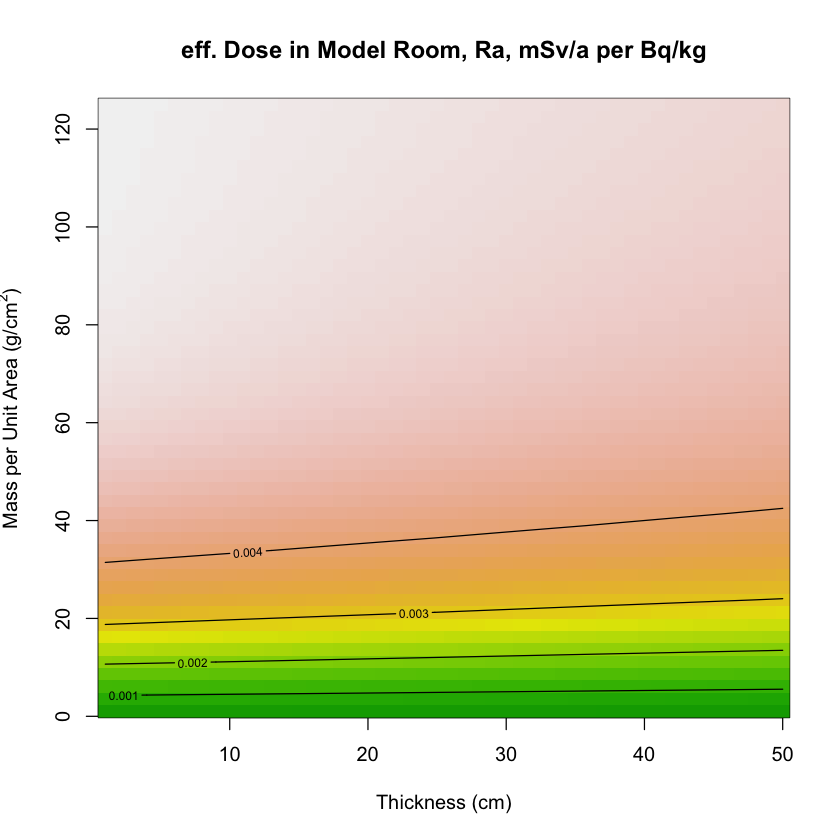In :
image(q1, q2, Th_FD, col = terrain.colors(256), axes = TRUE,
main = "eff. Dose in Model Room, Th, mSv/a per Bq/kg",
xlab = "Thickness (cm)", ylab=expression("Mass per Unit Area (g/cm"^2*")"))
contour(q1, q2, Th_FD, levels = c(0.001,0.002,0.003,0.004), add = TRUE)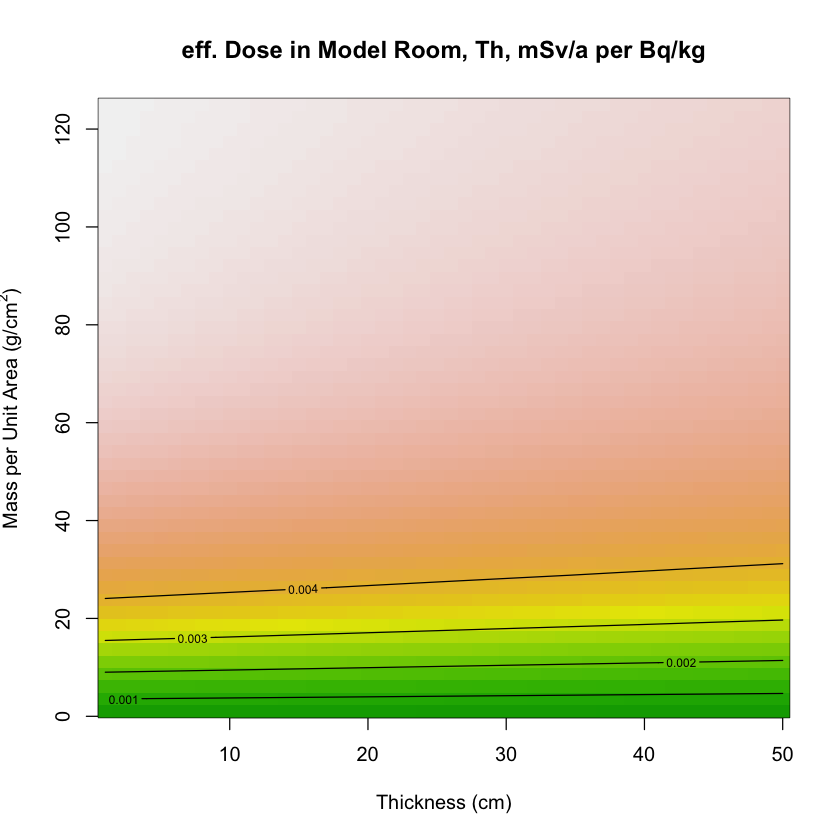In :
image(q1, q2, K_FD, col = terrain.colors(256), axes = TRUE,
main = "eff. Dose in Model Room, K, mSv/a per Bq/kg",
xlab = "Thickness (cm)", ylab=expression("Mass per Unit Area (g/cm"^2*")"))
contour(q1, q2, K_FD, levels = c(0.0001,0.0002,0.0003,0.0004), add = TRUE)